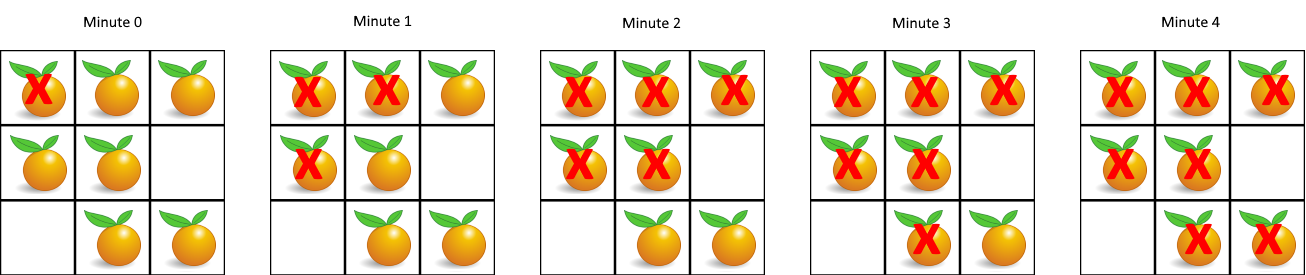994. Rotting Oranges

You are given an `m x n` `grid` where each cell can have one of three values:

• `0` representing an empty cell,
• `1` representing a fresh orange, or
• `2` representing a rotten orange.

Every minute, any fresh orange that is 4-directionally adjacent to a rotten orange becomes rotten.

Return the minimum number of minutes that must elapse until no cell has a fresh orange. If this is impossible, return `-1`.

Example 1:```Input: grid = [[2,1,1],[1,1,0],[0,1,1]]
Output: 4
```

Example 2:

```Input: grid = [[2,1,1],[0,1,1],[1,0,1]]
Output: -1
Explanation: The orange in the bottom left corner (row 2, column 0) is never rotten, because rotting only happens 4-directionally.
```

Example 3:

```Input: grid = [[0,2]]
Output: 0
Explanation: Since there are already no fresh oranges at minute 0, the answer is just 0.
```

Constraints:

• `m == grid.length`
• `n == grid[i].length`
• `1 <= m, n <= 10`
• `grid[i][j]` is `0`, `1`, or `2`.

994. Rotting Oranges
``````struct Solution;

use std::collections::VecDeque;

struct Orange {
r: usize,
c: usize,
t: i32,
}

impl Solution {
fn oranges_rotting(mut grid: Vec<Vec<i32>>) -> i32 {
let n = grid.len();
let m = grid.len();
let mut queue: VecDeque<Orange> = VecDeque::new();
for i in 0..n {
for j in 0..m {
if grid[i][j] == 2 {
queue.push_back(Orange { r: i, c: j, t: 0 });
}
}
}
let mut res = 0;
while let Some(o) = queue.pop_front() {
let r = o.r;
let c = o.c;
let t = o.t;
if r > 0 && grid[r - 1][c] == 1 {
grid[r - 1][c] = 2;
res = i32::max(res, t + 1);
queue.push_back(Orange {
r: r - 1,
c,
t: t + 1,
});
}
if r < n - 1 && grid[r + 1][c] == 1 {
grid[r + 1][c] = 2;
res = i32::max(res, t + 1);
queue.push_back(Orange {
r: r + 1,
c,
t: t + 1,
});
}
if c > 0 && grid[r][c - 1] == 1 {
grid[r][c - 1] = 2;
res = i32::max(res, t + 1);
queue.push_back(Orange {
r,
c: c - 1,
t: t + 1,
});
}
if c < m - 1 && grid[r][c + 1] == 1 {
grid[r][c + 1] = 2;
res = i32::max(res, t + 1);
queue.push_back(Orange {
r,
c: c + 1,
t: t + 1,
});
}
}
for i in 0..n {
for j in 0..m {
if grid[i][j] == 1 {
return -1;
}
}
}
res
}
}

#[test]
fn test() {
let grid: Vec<Vec<i32>> = vec_vec_i32![[2, 1, 1], [1, 1, 0], [0, 1, 1]];
assert_eq!(Solution::oranges_rotting(grid), 4);
let grid: Vec<Vec<i32>> = vec_vec_i32![[2, 1, 1], [0, 1, 1], [1, 0, 1]];
assert_eq!(Solution::oranges_rotting(grid), -1);
let grid: Vec<Vec<i32>> = vec_vec_i32![[0, 2]];
assert_eq!(Solution::oranges_rotting(grid), 0);
}
``````